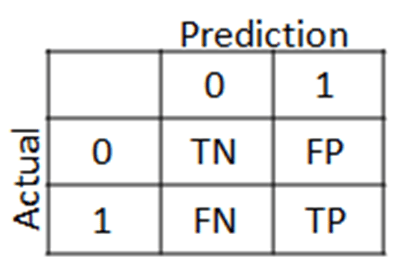# Using Confusion Matrices to Evaluate Performance

The data within a confusion matrix can be used to calculate several different statistics that can indicate the usefulness of a statistical model in machine learning. In this post, we willlook at several commonly used measures, specifically…

• accuracy
• error
• sensitivity
• specificity
• precision
• recall
• f-measure

Accuracy

Accuracy is probably the easiest statistic to understand. Accuracy is the total number of items correctly classified divided by the total number of items below is the equation

accuracy =   TP + TN
TP + TN + FP  + FN

TP =  true positive, TN =  true negative, FP = false positive, FN = false negative

Accuracy can range in value from 0-1 with one representing 100% accuracy. Normally, you don’t want perfect accuracy as this is an indication of overfitting and your model will probably not do well with other data.

Error

Error is the opposite of accuracy and represents the percentage of examples that are incorrectly classified its equation is as follows.

error =   FP + FN
TP + TN + FP  + FN

The lower the error the better in general. However, if an error is 0 it indicates overfitting. Keep in mind that error is the inverse of accuracy. As one increases the other decreases.

Sensitivity

Sensitivity is the proportion of true positives that were correctly classified.The formula is as follows

sensitivity =       TP
TP + FN

This may sound confusing but high sensitivity is useful for assessing a negative result. In other words, if I am testing people for a disease and my model has a high sensitivity. This means that the model is useful telling me a person does not have a disease.

Specificity

Specificity measures the proportion of negative examples that were correctly classified. The formula is below

specificity =       TN
TN + FP

Returning to the disease example, a high specificity is a good measure for determining if someone has a disease if they test positive for it. Remember that no test is foolproof and there are always false positives and negatives happening. The role of the researcher is to maximize the sensitivity or specificity based on the purpose of the model.

Precision

Precision is the proportion of examples that are really positive. The formula is as follows

precision =       TP
TP + FP

The more precise a model is the more trustworthy it is. In other words, high precision indicates that the results are relevant.

Recall

Recall is a measure of the completeness of the results of a model. It is calculated as follows

recall =       TP
TP + FN

This formula is the same as the formula for sensitivity. The difference is in the interpretation. High recall means that the results have a breadth to them such as in search engine results.

F-Measure

The f-measure uses recall and precision to develop another way to assess a model. The formula is below

sensitivity =      2 * TP
2 * TP + FP + FN

The f-measure can range from 0 – 1 and is useful for comparing several potential models using one convenient number.

Conclusion

This post provided a basic explanation of various statistics that can be used to determine the strength of a model. Through using a combination of statistics a researcher can develop insights into the strength of a model. The only mistake is relying exclusively on any single statistical measurement.